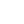# How to Get Current Month in Javascript

By Henri ParviainenIf you need a solution to get the current month value using Javascript, this is the post for you.

Getting the current month is very simple. We can take advantage of the `getMonth()` method in the Javascript `Date` object which returns the month number (index) of the date.

Note that the `getMonth()` returns the index of the month which starts from 0 so January = 0, February = 1 etc..

So, you need to add one to the value that `getMonth()` returns if you want to display the numeric month value.

However, if you need to do any time calculations or comparisons with dates, make sure to do them before you add one to the month value.

## Example for getting current month

``````const today = new Date();

let month = today.getMonth() + 1;

// month = 3 (current month value between 1-12)``````

In the code above we first get current date as a javascript date object. Then, we use the getMonth method to get current month index and increment it by one. The return value of the operation is a numeric value of the current month.

What if you want to get the name of the month in question? Read further to learn how to do just that.

## How to get month name from date

We can convert a date to the name of the month by using the `toLocaleString()` method in the `Date` object.

``````let today = new Date();

let thisMonth = today.toLocaleString("default", (month: "long"));

// thisMonth = 'March'``````

## How to get the previous month

If you want to get the previous month for a date, we can use the `setMonth()` method and subtract one from the current month.

Luckily Javascript will handle all the calculations for us so it will also work if the current month is January.

``````let today = new Date("2022-01-01");

today.setMonth(today.getMonth() - 1);

let previousMonth = today.toLocaleString("en-US", {
month: "long",
});

// previousMonth = "December"``````